# Practice Quiz in DC Biasing – FET Part 3

(Last Updated On: February 3, 2020)

This is the Online Practice Quiz in DC Biasing – FETs Part 3 from the book, Electronic Devices and Circuit Theory 10th Edition by Robert L. Boylestad. If you are looking for a reviewer in Electronics Engineering this will definitely help. I can assure you that this will be a great help in reviewing the book in preparation for your Board Exam. Make sure to familiarize each and every questions to increase the chance of passing the ECE Board Exam.

### Continue Part III of the Online Practice Quiz

#### Quiz in DC Biasing – FETs

Question 21. Which of the following current equations is true?

A. IG = ID

B. IG = IS

C. ID = IS

D. IG = ID = IS

Question 22. Given the values of VDQ and IDQ for this circuit, determine the required values of RD and RS.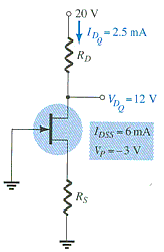A. 2 kΩ, 2 kΩ

B. 1 kΩ, 5.3 kΩ

C. 3.2 kΩ, 400 Ω

D. 2.5 kΩ, 5.3 kΩ

Question 23. For the noninverting amplifier, one of the most important advantages associated with using a JFET for control is the fact that it is _____ rather than _____ control.

A. dc, ac

B. ac, dc

Question 24. Which of the following describe(s) the difference(s) between JFETs and depletion-type MOSFETs?

A. VGS can be positive or negative for the depletion-type.

B. ID can exceed IDSS for the depletion-type.

C. The depletion-type can operate in the enhancement mode.

D. All of these

Question 25. Calculate VD.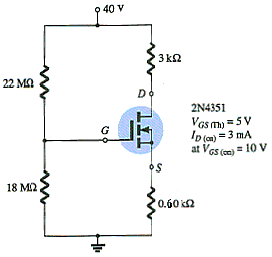A. 23.0 V

B. 17.0 V

C. 4.6 V

D. 12.4 V

Question 26. The self-bias configuration eliminates the need for two dc supplies.

A. True

B. False

Question 27. What are the voltages across RD and RS?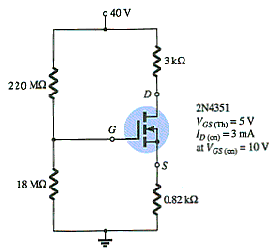A. 0 V, 0 V

B. 5 V, 5 V

C. 10 V, 10 V

D. 20 V, 20 V

Question 28. Calculate the value of RD’.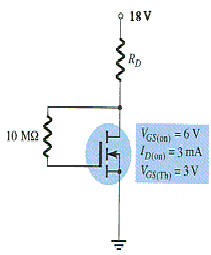A. 2 kΩ

B. 3 kΩ

C. 3.5 kΩ

D. 4.13 kΩ

Question 29. Determine the value of VDSQ.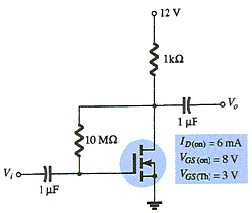A. 3.5 V

B. 4.86 V

C. 4.86 V

D. 10 V

Question 30. What is the new value of RD when there is 7 V across VDS?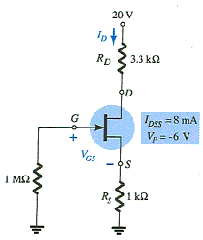A. 3 kΩ

B. 3.3 kΩ

C. 4 kΩ

D. 5 kΩ

### More Practice Quiz in DC Biasing – FETs

Practice Quiz Part 1

Practice Quiz Part 2

Practice Quiz Part 3

Practice Quiz Part 4

Practice Quiz Part 5

Practice Quiz Part 6

### See: Complete List of Practice Quizzes

Note: After taking this particular quiz, you can proceed to check all the topics.